# Geometric progression - high school - math problems

#### Number of problems found: 112

• Geometric progression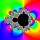Fill 4 numbers between 4 and -12500 to form geometric progression.
• Geometric progressionIn geometric progression, a1 = 7, q = 5. Find the condition for n to sum first n members is: sn≤217.
• Geometric progression 2There is geometric sequence with a1=5.7 and quotient q=-2.5. Calculate a17.
• Geometric seqFind the third member of geometric progression if a1 + a2 = 36 and a1 + a3 = 90. Calculate its quotient.
• GP - 8 itemsDetermine the first eight members of a geometric progression if a9=512, q=2
• Missing term 2What is the missing term for the Geometric Progression (GP) 3, 15, 75,__, 1875?
• Q of GP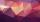Calculate quotient of geometric progression if a1=5 a a1+a2=12.
• Sum 1-6Find the sum of the geometric progression 3, 15, 75,… to six terms.
• Geometric progressiobIf the sum of four consective terms of geometric progression is 80 and arithmetic mean of second and fourth term is 30 then find terms?
• GP - three membersThe second and third of a geometric progression are 24 and 12(c+1) respectively, given that the sum of the first three terms of progression is 76 determine value of c
• Geometric sequence 5About members of geometric sequence we know: ? ? Calculate a1 (first member) and q (common ratio or q-coefficient)
• Geometric sequenceIn the geometric sequence is a4 = 20 a9 = -160. Calculate the first member a1 and quotient q.
• Quotient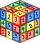Determine the quotient and the second member of the geometric progression where a3=10, a1+a2=-1,6 a1-a2=2,4.
• Six speeds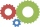A drilling machine is to have 6 speed ranging from 50 to 750 revolution per minute. If the speed forms a geometric progression, determine their values.
• Gp - 80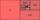Sum of the first four members of a geometric progression is 80. Determine they if we know that the fourth member is nine times greater than the second.
• Three members GPThe sum of three numbers in GP (geometric progression) is 21 and the sum of their squares is 189. Find the numbers.
• Geometric progression 48,4√2,4,2√2
• Insert into GPBetween numbers 5 and 640 insert as many numbers to form geometric progression so sum of the numbers you entered will be 630. How many numbers you must insert?
• Geometric sequence 3In geometric sequence is a8 = 312500; a11= 39062500; sn=1953124. Calculate the first item a1, quotient q and n - number of members by their sum s_n.
• Tenth memberCalculate the tenth member of geometric sequence when given: a1=1/2 and q=2

Do you have an interesting mathematical word problem that you can't solve it? Submit a math problem, and we can try to solve it.

We will send a solution to your e-mail address. Solved examples are also published here. Please enter the e-mail correctly and check whether you don't have a full mailbox.

Please do not submit problems from current active competitions such as Mathematical Olympiad, correspondence seminars etc...

Geometric progression - math word problems. Examples for secondary school students.